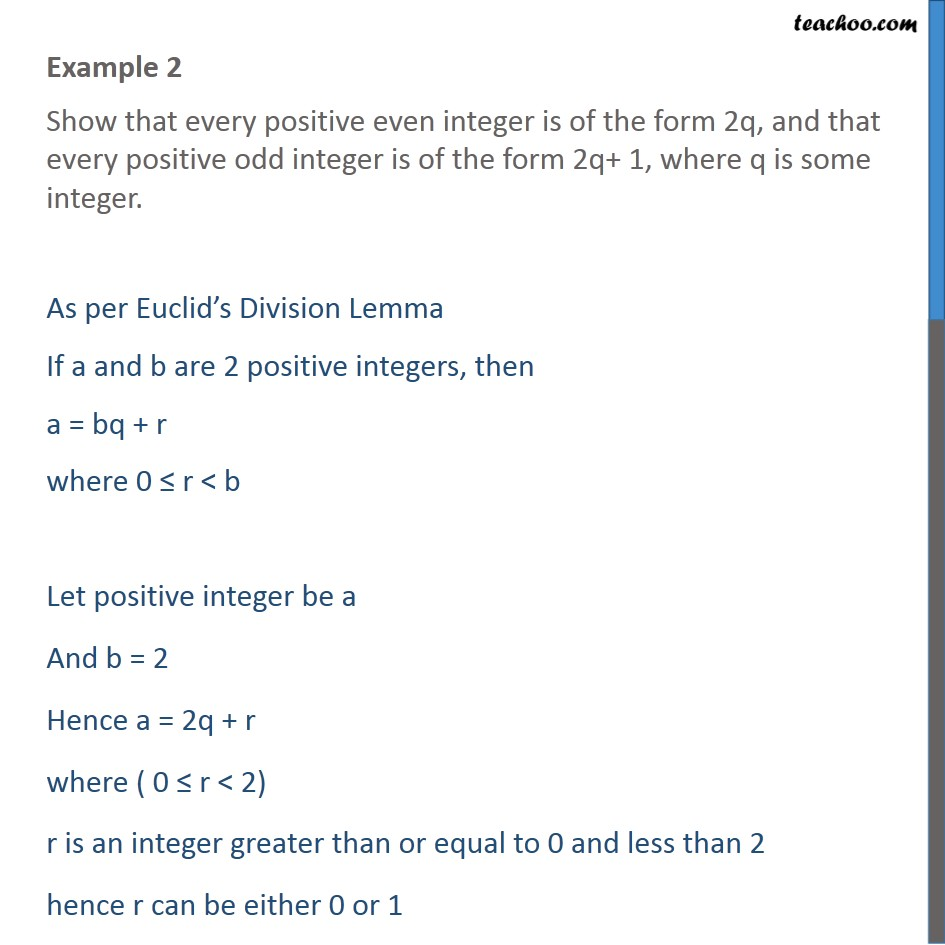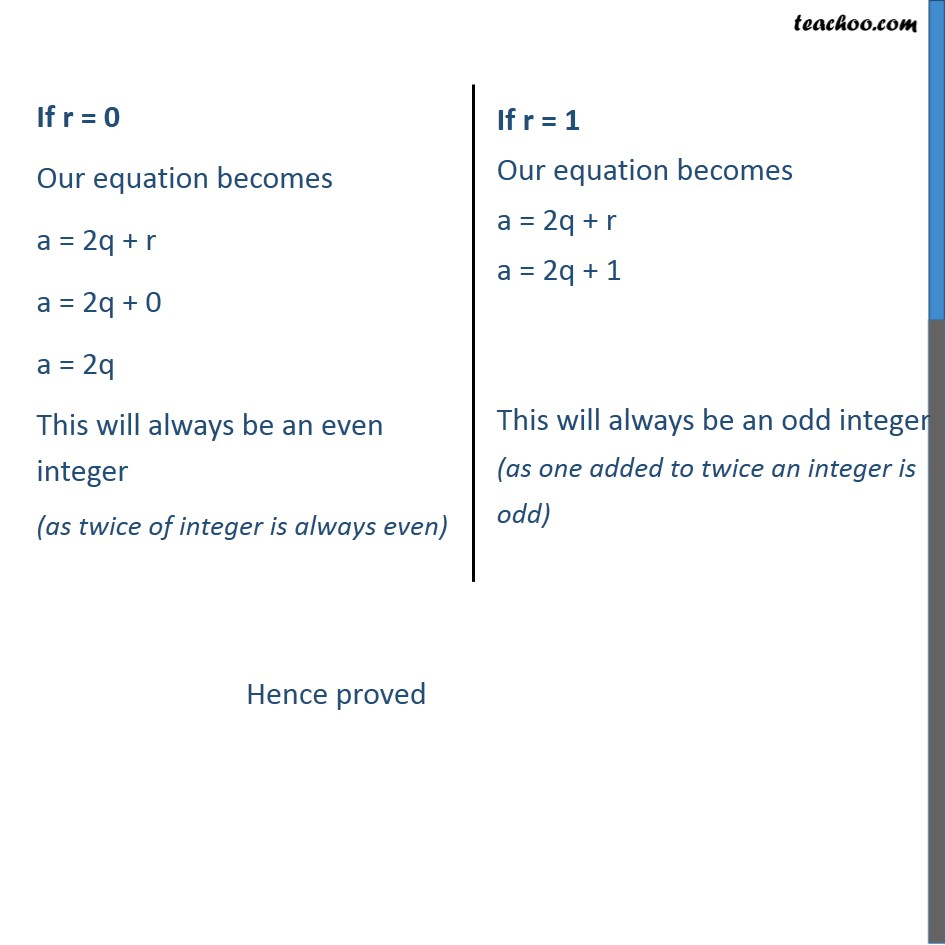Check Important Questions and Chapter Summary -https://you.tube/Real-Numbers-Class-101. Chapter 1 Class 10 Real Numbers
2. Concept wise
3. Euclid's Division Algorithm - Proving

Transcript

Example 2 Show that every positive even integer is of the form 2q, and that every positive odd integer is of the form 2q+ 1, where q is some integer. As per Euclid’s Division Lemma If a and b are 2 positive integers, then a = bq + r where 0 ≤ r < b Let positive integer be a And b = 2 Hence a = 2q + r where ( 0 ≤ r < 2) r is an integer greater than or equal to 0 and less than 2 hence r can be either 0 or 1

Euclid's Division Algorithm - Proving